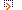# Differential Equations DirectoryFeatured Listings

Navier-Stokes Type Equations
Explicit solutions provided for this particular type of equation and their relations to the heat equation, Burger's equation, and Euler's equation.
Keywords: burger's_equation , explicit_solutions_provided , fluid_mechanics
www.coolissues.com/mathematics/Navier-Stokes/nstokes.htm

Sturm-Liouville Theory
Sturm-Liouville Theory
www.math.ohio-state.edu/~gerlach/math/BVtypset/node59.html

A web text on the background to the extrapolation method for the numerical solution of elliptic boundary value problems by Kwok Sui-Yuen Billy.
www.sci.hkbu.edu.hk/mscsc/dissert/billy/node1.html

Site Listings

Navier-Stokes Type Equations
Explicit solutions provided for this particular type of equation and their relations to the heat equation, Burger's equation, and Euler's equation.
Keywords: burger's_equation , explicit_solutions_provided , fluid_mechanics
www.coolissues.com/mathematics/Navier-Stokes/nstokes.htm

Sturm-Liouville Theory
Sturm-Liouville Theory
www.math.ohio-state.edu/~gerlach/math/BVtypset/node59.html